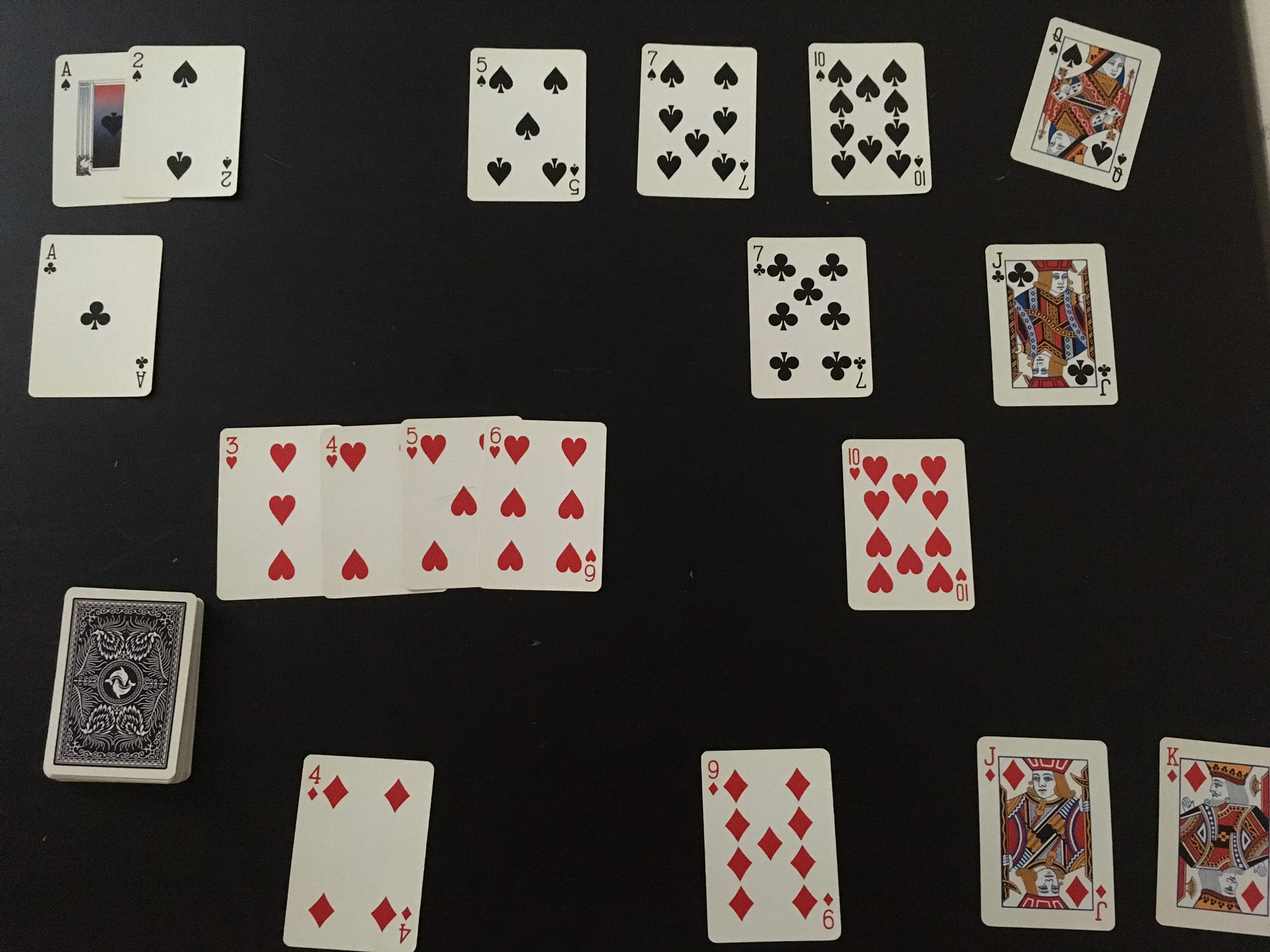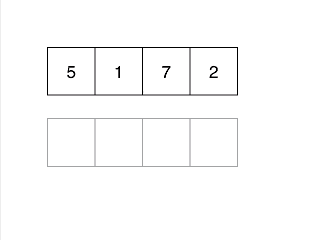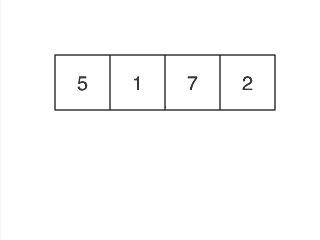# Insertion Sort

This is part of the Algorithms with Auntie Aja series.

This is part of my series on algorithms and data structures called “Algorithms with Auntie Aja”. This series provides an introduction to data structures and algorithms for folks who never learned them or have forgotten them.

### Algorithms with Auntie Aja: Sorting

Sorting algorithms are a large part of any course in algorithms. They also frequently show up in interviews. In my not so humble opinion, the correct answer when anyone asks you to sort something in an interview is to use whatever sort is built in to your language. Sorting is deceptively hard. Many folks think it should be easy. After all 2 and 3 year-olds can sort things. It turns out that there are a lot of subtle things to consider when writing a sorting algorithm. Off-by-one errors (fence post errors) are one common example.

### Insertion Sort

Insertion sort is the first sort I’m going to cover. There are some simpler sorting algorithms (like bubble sort) but insertion sort is simple to explain. You likely use a method similar to insertion sort when you sort things in every day life. Additionally, it performs well enough on small data sets and you don’t have to have all the items up front. You can put new items in the correct place as they become available. This makes it an online sorting algorithm.

The setup for insertion sort is simple. You have two collections. One contains the unsorted items. The other contains the items that are sorted. If this is confusing, think about ordering a deck of cards. The shuffled (unsorted) cards are in the deck and the ones you’ve put in order are spread out on the table.When you start, the collection of sorted items is empty. To sort you look at the first item in the unsorted collection and put it in the proper place in the sorted collection. Then you put the next item in place. Repeat until sorted.### The Code

In the spirit of TDD I’m starting with three basic tests. Sorting an empty array, sorting an array of length one, and sorting an array with more than one element.

 ```1 2 3 4 5 6 7 8 9 10 11 12 13 14 15 16 17 ``` ```# test_insertion_sort.rb require 'minitest/autorun' require './insertion_sort' class TestInsertionSort < Minitest::Test def test_sort_empty_list assert_equal [], [].insertion_sort end def test_sort_list_length_one assert_equal , .insertion_sort end def test_sort_random_list assert_equal [1, 2, 3, 4, 5], [3, 2, 5, 1, 4].insertion_sort end end ```

Here’s a basic implementation of insertion sort. I’m monkey patching (re-opening) the Array class because my tests call insertion sort on an array.

 ```1 2 3 4 5 6 7 8 9 10 11 12 13 14 15 16 17 18 19 ``` ```# insertion_sort.rb class Array def insertion_sort sorted = [] self.each do |item| (0..sorted.length).each do |index| if sorted[index].nil? then sorted.insert index, item elsif sorted[index] > item then sorted.insert index, item break end end end sorted end end ```

There’s a fair amount going on in those 18 lines. On line 3 I create a new array called `sorted` that will hold the sorted results (the ones on the table from the cards analogy). Next (line 5) I iterate through all the elements of `self` which is the unsorted array.

Lines 6 - 13 look through the sorted array and try to find the first element that is greater than the item I’m currently looking at. Once it is found (line 9), I use `Array#insert` which inserts an item in an array in front of the specified index.

If the item I’m trying to find is greater than all the other elements in the sorted array, index will eventually be `sorted.length`. This is because I’m using two dots for the range. Two dots means both ends of the range are included. If index is `'sorted.length` then `sorted[index]` will be nil. I catch that case on line 8 and use insert to add the item to the end of the sorted array.

### Sorting In Place

The version of insertion sort above works but makes a copy of the array and leaves the original untouched. If I want to sort my original array I can write `#insertion_sort!`. Here are the new tests:

 ```1 2 3 4 5 6 7 8 9 10 11 12 13 14 15 16 17 18 ``` ```#test_insertion_sort.rb def test_sort_bang_empty_list ary = [] ary.insertion_sort! assert_equal [], ary end def test_sort_bang_list_length_one ary =  ary.insertion_sort! assert_equal , ary end def test_sort_bang_random_list ary = [3, 2, 5, 1, 4] ary.insertion_sort! assert_equal [1, 2, 3, 4, 5], ary end ```

Since I’m testing a method that modifies the array I need to assign the array, call my method, and then assert it did the correct thing. That’s why these tests are longer than the tests for `insertion_sort` above.

Here’s the code:

 ```1 2 3 4 5 6 7 8 9 10 ``` ```# insertion_sort.rb def insertion_sort! (1...self.length).each do |i| j = i while j > 0 and self[j - 1] > self[j] self[j], self[j - 1] = self[j - 1], self[j] j -= 1 end end end ```

This version is much shorter. Starting with the second element of the array (index 1) I take that item and compare it to all the items before it in the array. When I find one that is smaller I insert the current item right after it. If I make it all the way to the front the current item must be the smallest so I put the current item at the front of the array.### Conclusion

Insertion sort isn’t a particularly efficient algorithm but it is easy to understand and relatively straightforward to write up. There are a handful of different ways for implementing it and I encourage you to take the tests I provided and try to find a different way to implement it.

Code used in this post is available on GitHub.